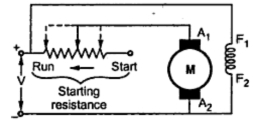### Necessity of Starter for D.C. Motors

All the d.c. motor are basically self starting motors. Whenever the armature and the field winding of a d.c. motor receives supply, motoring action takes place. So d.c. motors do not require any additional device to start it. The device to be used as a starter conveys a wrong meaning.
Note : So starter is not required to start a d.c. motor but it enables us to start the motor in a desired, safe way.
Now at the starting instant the speed of the motor is zero, (N = 0). As speed is zero, there can not be any back e.m.f. as Eb α  N and N is zero at start.
...         Eb    at start = 0
The voltage equation of a d.c. motor is,
V = Eb + Ia Ra
So at start,   V =  Ia Ra                                                       as E= 0
...           Ia = V/Ra                                                         .............. at start
Note : Generally motor is switched on with normal voltage and as armature resistance is very small, the armature current at start is very high.
Consider a motor having full load input power as 8000 watts. The motor rated voltage be 250 V and armature resistance is 0.5 Ω.
Then at start, Eb = 0 and motor is operated at 250 V supply, so
Ia = V/Ra   = 250/0.5 = 500 A
while its full load current can be calculated as,
IFull load  = Power input on full load/Supply voltage   = 8000/250 = 32 A
So at start, motor is showing a tendency to draw an armature current which is 15 to 20 times more than the full load current.
Such high current drawn by the armature at start is highly objectionable for the following reasons :
1. In a constant voltage system, such high inrush of current may cause tremendous line voltage fluctuations. This may affect the performance of the other equipments connected to the same line.
2. Such excessively high armature current, blows out the fuses.
3. If motor fails to start due to some problems with the field winding, then a large armature current flowing for a longer time may burn the insulation of the armature winding.
4. As the starting armature current is 10 to 15 times more than the full load current, the torque developed which is proportional to the Ia will also be 10 to 15 times, assuming shunt motor operation. So due to such high torque, the shaft and other accessories are thus subjected to large mechanical stresses. These stresses may cause permanent mechanical damage to the motor.
To restrict this high starting armature current, a variable resistance is connected in series with the armature at start. This resistance is called starter or a starting resistance. So starter is basically a current limiting device. In the beginning the entire resistance is in the series with the armature and then gradually cut off as motor gathers speed, producing the back e.m.f. The basic arrangement is shown in the Fig. 1.Fig. 1 Basic arrangement of a starter
In addition to the starting resistance, there are some protective devices provided in a starter. There are two types of starters used for d.c. shunt motors.
a) Three point starter
b) Four point starter

1.